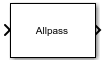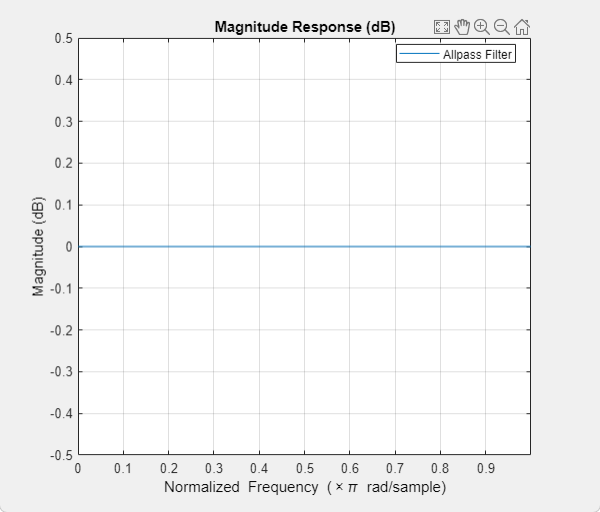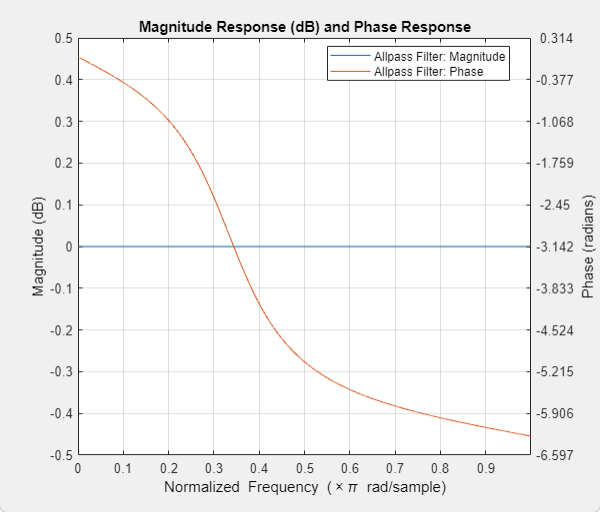# Allpass Filter

Single-section or multiple-section allpass filter

• Library:
• DSP System Toolbox / Filtering / Filter Implementations

•## Description

The Allpass Filter block filters each channel of the input signal independently using a single-section or multiple-section (cascaded) allpass filter. You can implement the allpass filter using a minimum multiplier, wave digital filter, or a lattice structure.

In minimum multiplier form, the block uses the minimum number of required multipliers, n, with 2n delay units and 2n adders. In wave digital filter form, the block uses only n multipliers and n delay units, at the expense of 3n adders. The lattice structure uses 2n multipliers, n delay units, and 2n adders. For more details on these structures, see Algorithms.

## Ports

### Input

expand all

Input data that is passed into the allpass filter. The block accepts real-valued or complex-valued multichannel inputs, that is, m-by-n size inputs, where m ≥ 1 and n ≥ 1. The block also accepts variable-size inputs. That is, you can change the size of each input channel during simulation. However, the number of channels cannot change.

This port is unnamed until you set Internal allpass structure to ```Minimum multiplier``` or `Lattice`, and select the Specify coefficients from input port parameter.

Data Types: `single` | `double`
Complex Number Support: Yes

This port inputs the coefficients of the allpass filter. When you set Internal allpass structure to `Minimum multiplier`, the coeffs port accepts matrices of size N-by-1 or N-by-2. When you set Internal allpass structure to `Lattice`, the coeffs port accepts an N-by-1 column vector or an 1-by-N row vector.

#### Dependencies

This port appears when you set Internal allpass structure to ```Minimum multiplier``` or `Lattice`, and select the Specify coefficients from input port parameter.

Data Types: `single` | `double` | `int8` | `int16` | `int32` | `uint8` | `uint16` | `uint32`

### Output

expand all

The size of the filtered output matches the size of the input.

Data Types: `single` | `double`
Complex Number Support: Yes

## Parameters

expand all

If a parameter is listed as tunable, then you can change its value during simulation.

• `Minimum multiplier` — This structure uses the minimum number of required multipliers, n, with 2n delay units and 2n adders. The coefficients to this structure are specified through the Allpass polynomial coefficients parameter.

• `Wave Digital Filter` — The structure uses n multipliers and n delay units, at the expense of 3n adders. The coefficients to this structure are specified through the Wave Digital Filter allpass coefficients parameter.

• `Lattice` — The structure uses 2n multipliers, n delay units, and 2n adders. The coefficients to this structure are specified through the Lattice allpass coefficients parameter.

For more details on these structures, see Algorithms.

When you select this check box and set Internal allpass structure to `Minimum multiplier`, the allpass polynomial coefficients are input through the coeffs port. When you clear this check box, the allpass polynomial coefficients are specified on the block dialog through the Allpass polynomial coefficients parameter.

When you select this check box and set Internal allpass structure to `Lattice`, the lattice allpass coefficients are input through the coeffs port. When you clear this check box, the lattice allpass coefficients are specified on the block dialog through the Lattice allpass coefficients parameter.

#### Dependencies

This parameter applies when you set Internal allpass structure to ```Minimum multiplier``` or `Lattice`.

Specify the real allpass polynomial filter coefficients in minimum multiplier form as an N-by-1 matrix or an N-by-2 matrix.

• N-by-1 matrix — The block realizes N first-order allpass sections.

• N-by-2 matrix — The block realizes N second-order allpass sections.

The default value, `[ -2^(-1/2), 1/2 ]`, defines a stable second-order allpass filter with poles and zeros at ±π/3 in the z-plane.

Tunable: Yes

#### Dependencies

To enable this parameter, set Internal allpass structure to ```Minimum multiplier``` and clear the Specify coefficients from input port parameter.

Specify the real allpass filter coefficients in wave digital filter form. The coefficients can be N-by-1 matrix for N first-order allpass sections and N-by-2 matrix for N second-order allpass sections. The default value, `[1/2, -2^(1/2)/3]`, is a transformed version of the default value of allpass polynomial coefficients. This value is computed using ```allpass2wdf(Allpass polynomial coefficients)```. These coefficients define the same stable second-order allpass filter as when the allpass structure is set to `Minimum multiplier`.

Tunable: Yes

#### Dependencies

To enable this parameter, set Internal allpass structure to ```Wave Digital Filter```.

• on — When you set select this check box, the last section is considered first order. Also, the second element of the last row of the N-by-2 matrix is ignored.

• off — When you do not select this check box, the last section is considered second-order.

#### Dependencies

To enable this parameter, set Internal allpass structure to ```Minimum multiplier``` or ```Wave Digital Filter```.

Specify the real or complex allpass coefficients as lattice reflection coefficients. The default value, `[-2^(1/2)/3, 1/2]`, is a transformed and transposed version of the default value of the allpass polynomial coefficients. This value is computed using `transpose(tf2latc(1, [1 A]))`, where A is the value specified in Allpass polynomial coefficients.

Tunable: Yes

#### Dependencies

To enable this parameter, set Internal allpass structure to `Lattice` and clear the Specify coefficients from input port parameter.

Opens the Filter Visualization Tool, `fvtool`, and displays the magnitude response of the allpass filter. The response is based on the parameters. Changes made to these parameters update `fvtool`.To update the magnitude response while `fvtool` is running, modify the block parameters and click .

To view the magnitude response and phase response simultaneously, click the button on the toolbar.In this example, the magnitude response is flat and the phase response varies with frequencies. This varying phase response has applications in phase equalization, notch filtering, and multirate filtering. You can realize a lowpass filter using a parallel combination of two allpass filters that have 180 degrees of phase shift with respect to each other.

• `Code generation`

Simulate model using generated C code. The first time you run a simulation, Simulink® generates C code for the block. The C code is reused for subsequent simulations, as long as the model does not change. This option requires additional startup time but provides faster simulation speed than ```Interpreted execution```.

• `Interpreted execution`

Simulate model using the MATLAB®  interpreter. This option shortens startup time but has slower simulation speed than ```Code generation```.

## Block Characteristics

 Data Types `double` | `single` Multidimensional Signals `No` Variable-Size Signals `Yes`

## Algorithms

expand all

The transfer function of an allpass filter is given by

$H\left(z\right)=\frac{c\left(n\right)+c\left(n-1\right){z}^{-1}+...+{z}^{-n}}{1+c\left(1\right){z}^{-1}+...+c\left(n\right){z}^{-n}}$.

c is allpass polynomial coefficients vector. The order, n, of the transfer function is the length of vector c.

In the minimum multiplier form and wave digital form, the allpass filter is implemented as a cascade of either second-order (biquad) sections or first-order sections. When the coefficients are specified as an N-by-2 matrix, each row of the matrix specifies the coefficients of a second-order filter. The last element of the last row can be ignored based on the trailing first-order setting. When the coefficients are specified as an N-by-1 matrix, each element in the matrix specifies the coefficient of a first-order filter. The cascade of all the filter sections forms the allpass filter.

In the lattice form, the coefficients are specified as a vector.

These structures are computationally more economical and structurally more stable compared to the generic IIR filters, such as df1, df1t, df2, df2t. For all structures, the allpass filter can be a single-section or a multiple-section (cascaded) filter. The different sections can have different orders, but they are all implemented according to the same structure.

 Regalia, Philip A., Sanjit K. Mitra, and P.P.Vaidyanathan. “The Digital All-Pass Filter: A Versatile Signal Processing Building Block.” Proceedings of the IEEE. 76, no. 1 (1988): 19–37.

 Lutovac, M., D. Tosic, and B. Evans. Filter Design for Signal Processing Using MATLAB and Mathematica. Upper Saddle River, NJ: Prentice Hall, 2001.

## SupportGet trial now